How to Create simulated data for classification in Python?

• Last Updated : 17 Oct, 2021

In this article, we are going to see how to create simulated data for classification in Python.

We will use the sklearn library that provides various generators for simulating classification data.

Single Label Classification

Here we are going to see single-label classification, for this we will use some visualization techniques.

Example 1: Using make_circles()

make_circles generates 2d binary classification data with a spherical decision boundary.

Python3

 from sklearn.datasets import make_circlesimport pandas as pdimport matplotlib.pyplot as plt  X, y = make_circles(n_samples=200, shuffle=True,                     noise=0.1, random_state=42)plt.scatter(X[:, 0], X[:, 1], c=y)plt.show()

Output: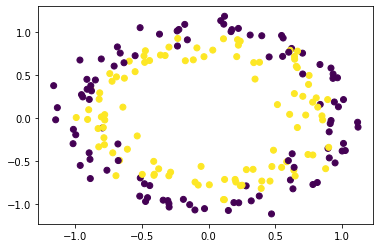Example 2: Using make_moons()

make_moons() generates 2d binary classification data in the shape of two interleaving half circles.

Python3

 from sklearn.datasets import make_moonsimport pandas as pdimport matplotlib.pyplot as plt  X, y = make_moons(n_samples=200, shuffle=True,                  noise=0.15, random_state=42)plt.scatter(X[:, 0], X[:, 1], c=y)plt.show()

Output: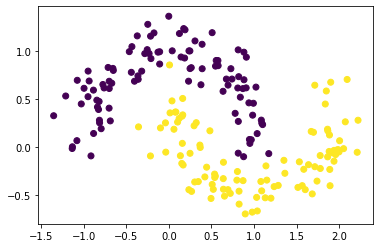Example 3. Using make_blobs()

make_blobs() generates data in form of blobs that can be used for clustering

Python3

 from sklearn.datasets import make_blobsimport pandas as pdimport matplotlib.pyplot as plt  X, y = make_blobs(n_samples=200, n_features=2, centers=3,                  shuffle=True, random_state=42)plt.scatter(X[:, 0], X[:, 1], c=y)plt.show()

Output: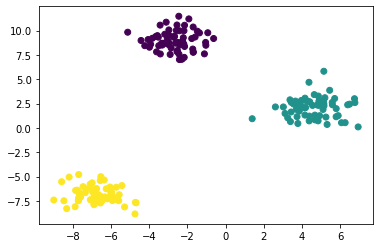Example 4. Using make_classification()

make_classification() generates a random n-class classification problem

Python3

 from sklearn.datasets import make_classificationimport pandas as pdimport matplotlib.pyplot as plt  X, y = make_classification(n_samples=100, n_features=5,                           n_classes=2,                           n_informative=2, n_redundant=2,                           n_repeated=0,                           shuffle=True, random_state=42)pd.concat([pd.DataFrame(X), pd.DataFrame(    y, columns=['Label'])], axis=1)

Output: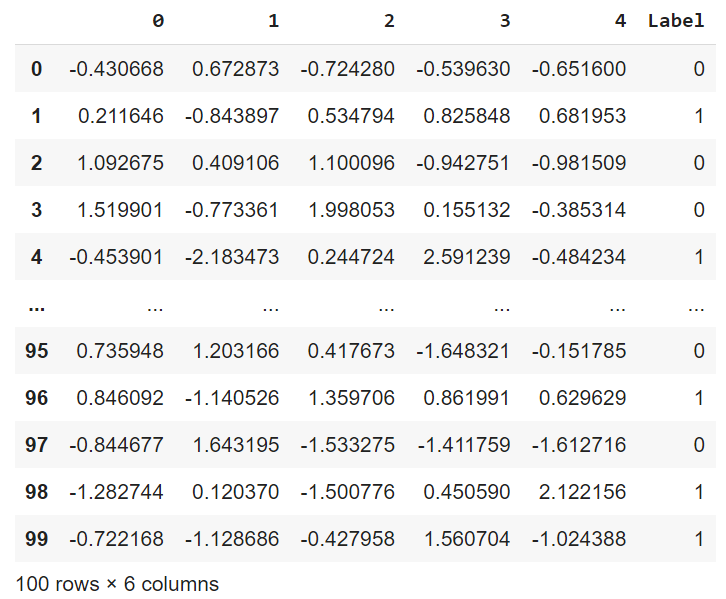Multi-Label Classification

make_multilabel_classification() generates a random multi-label classification problem.

Python3

 from sklearn.datasets import make_multilabel_classificationimport pandas as pdimport matplotlib.pyplot as plt  X, y = make_multilabel_classification(n_samples=100, n_features=5,                                       n_classes=2, n_labels=1,                                      allow_unlabeled=False,                                      random_state=42)pd.concat([pd.DataFrame(X), pd.DataFrame(y,                                          columns=['L1', 'L2'])],          axis=1)

Output: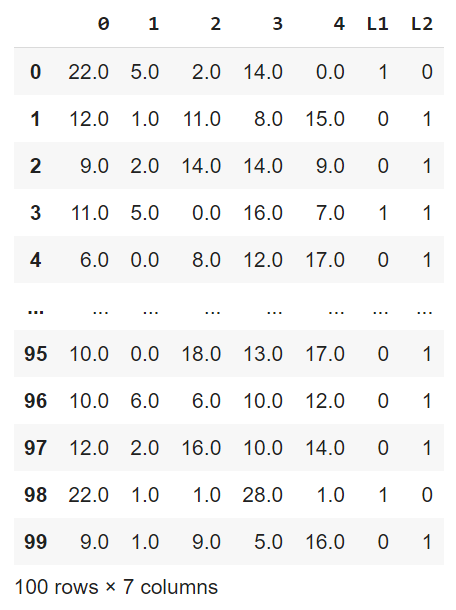Attention reader! Don’t stop learning now. Get hold of all the important Machine Learning Concepts with the Machine Learning Foundation Course at a student-friendly price and become industry ready.

My Personal Notes arrow_drop_up